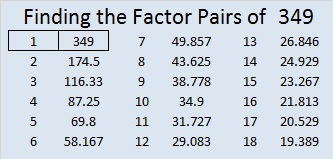# 349 and Level 6

When 2^349 is divided by 349, the remainder is 2, so 349 is VERY LIKELY a prime number. Scroll down past the puzzle to know for sure.Print the puzzles or type the factors on this excel file: 10 Factors 2015-01-05

• 349 is a prime number.
• Prime factorization: 349 is prime.
• The exponent of prime number 349 is 1. Adding 1 to that exponent we get (1 + 1) = 2. Therefore 349 has exactly 2 factors.
• Factors of 349: 1, 349
• Factor pairs: 349 = 1 x 349
• 349 has no square factors that allow its square root to be simplified. √349 ≈ 18.682

How do we know that 349 is a prime number? If 349 were not a prime number, then it would be divisible by at least one prime number less than or equal to √349 ≈ 18.682. Since 349 cannot be divided evenly by 2, 3, 5, 7, 11, 13, or 17, we know that 349 is a prime number.Prime Number Trivia: There are sixteen prime numbers between 300 and 400. Of those, 349 is the only one that doesn’t produce another prime number when 300 is subtracted from it. 349 – 300 = 49, a composite number. This piece of trivia might be helpful if you wanted to memorize all the prime numbers less than 1000.This site uses Akismet to reduce spam. Learn how your comment data is processed.